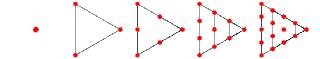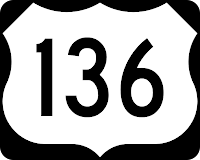## Tuesday, September 30, 2008

### 136

136 = 23 x 17.

136 is the smallest number (except 1) such that the sum of the cubes of the digits of the sum of the cubes of its digits equals itself: 13 + 33 + 63 = 244 and 23 + 43 + 43 = 136.

136 is a refactorable number (divisible by the count of its divisors); it has a total of 8 divisors (1, 2, 4, 8, 17, 34, 68, 136), with 8 among them.

136 is a triangular number, which can be represented in the form of a triangular grid of points, where the first row contains a single element and each subsequent row contains one more element than the previous one. Hence, it is the sum of the first 16 positive integers.A representation of the first few triangular numbers.
136 is 10001000 in base 2 (binary) and 88 in base 16.
136 has a representation as a sum of two squares: 136 = 62 + 102.136 Austria is an asteroid discovered in 1874.